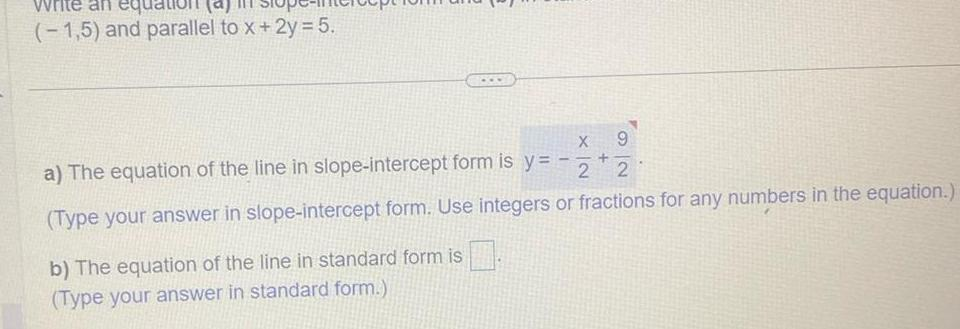Question:

# 1 5 and parallel to x 2y 5 X 9 a The equation of the line in

Last updated: 9/18/20231 5 and parallel to x 2y 5 X 9 a The equation of the line in slope intercept form is y 2 2 Type your answer in slope intercept form Use integers or fractions for any numbers in the equation b The equation of the line in standard form is Type your answer in standard form